Courses

# Soil Mechanics - 4

## 10 Questions MCQ Test GATE Civil Engineering (CE) 2022 Mock Test Series | Soil Mechanics - 4

Description
This mock test of Soil Mechanics - 4 for Civil Engineering (CE) helps you for every Civil Engineering (CE) entrance exam. This contains 10 Multiple Choice Questions for Civil Engineering (CE) Soil Mechanics - 4 (mcq) to study with solutions a complete question bank. The solved questions answers in this Soil Mechanics - 4 quiz give you a good mix of easy questions and tough questions. Civil Engineering (CE) students definitely take this Soil Mechanics - 4 exercise for a better result in the exam. You can find other Soil Mechanics - 4 extra questions, long questions & short questions for Civil Engineering (CE) on EduRev as well by searching above.
QUESTION: 1

### Piping in soil occurs when:

Solution:

The design of an embankment dam and other hydraulic structures, the choice of soils are aimed at reducing or eliminating the detrimental effects of seeping water. Where high hydraulic gradients exist there is a possibility that the seeping water may cause internal erosion within the dam, especially if the soil is poorly compacted. Erosion can work its way back into the embankment, creating voids in the form of channels or ‘pipes’, and thus impairing the stability of the dam. This form of erosion is referred to as piping and occurs when water flow upwards resulting in zero effective pressure.

QUESTION: 2

### The yield of a well depends upon:

Solution:

Yield of well means the discharge from the well excavated through given aquifer

Q = nva A = KiA

where

n is porosity

va is actual flow velocity of ground water

A is area of the aquifer opening onto the wells

K is permeability of soil

QUESTION: 3

### Consider the following statement with regard to prevention of formation of Quick sand Condition in the field? A. By lowering of the water table at the site before excavation at the excavation location B. By providing a sheet pile wall so as to decrease the upward flow length C. Application of surcharge (i.e. additional weight on the excavation side to increase the net weight of the soil mass. Identify the correct statements:

Solution:

Quick sand condition can be prevented by lowering of water table at site before excavation.

• It can also be prevented by increasing the upward flow length by providing sheet pile wall.
• Another method adopted at sites to avoid quick sand Condition is addition of surcharge (i.e additional weight) on the excavation side to increase the net weight of the soil mass.
*Answer can only contain numeric values
QUESTION: 4

Calculate the saturated unit weight (kN/m3)  of the soil sample for the Quick sand condition, if specify Gravity and void ratio of soil sample is  2.62 and 0.61 respectively. Take γW = 10 kN/m3

Solution:

For a Quicksand Condition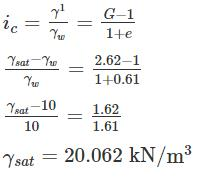QUESTION: 5

A series of wells were dug in a confined aquifer. The surface obtained by connecting the static water levels would be called:

Solution:

Confined aquifers are permeable rock units that are usually deeper under the ground than unconfined aquifers. They are overlain by relatively impermeable rock or clay that limits groundwater movement into, or out of, the confined aquifer. Groundwater in a confined aquifer is under pressure and will rise up inside a borehole drilled into the aquifer. The level to which the water rises is called the piezometric surface.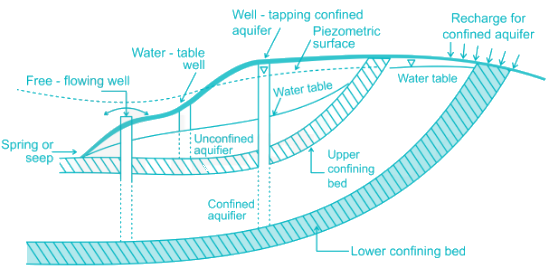QUESTION: 6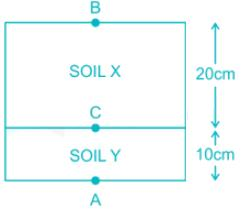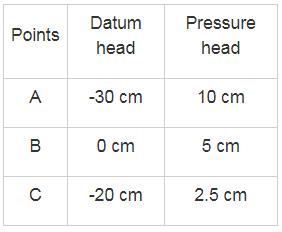Permeability of soil X is 3 × 10-4 cm/sec. What will be the permeability of Soil Y. Area of the cross-section for both the soil is the same.

Solution: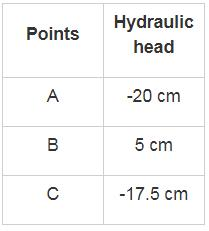(hL)x + (hL)y = Total head loss

Total head loss from B to A = - 20 - 5

= - 25 cm

or H = 25 cm

(hL)x = 5 - [-17.5] = - 22.5 cm

or (hL)x = 22.5 cm

(hL)y = 25 - 22.5 cm

(hL)y = 2.5 cm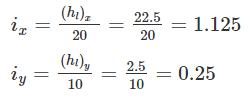Vx = Vy

Kix = Kiy

3 × 10-4 × 1.125 = Ky × 0.25

ky = 13.5 × 10-4 cm/sec

QUESTION: 7

An aquifer confined at top and bottom by impervious layers is stratified into three layers as follows: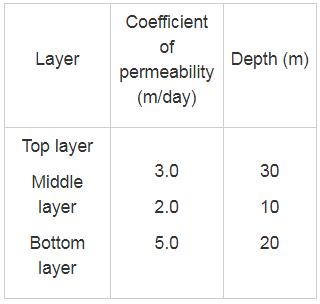The transmissibility of the aquifer in m2/day is

Solution:

An aquifer of unit width and thickness B carries a discharge = KB; where K is the co-efficient of permeability and B is the width. This discharge is termed as transmissibility T and has dimension L2T.

The transmissibility of a stratified formation = Ke∑B
Here K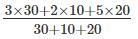3.5m/day
Transmissibility = 3.5 x 60 = 210 m2/day

*Answer can only contain numeric values
QUESTION: 8

Previous sand having air content 15% is used in the foundation of a masonry dam. If the maximum permissible upward gradient for a factor of safety of 3 against boiling is 0.315. The percentage of air voids in the previous sand will be ______ %.

Solution:

F.O.S against boiling is giving by

F.O.S =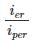ier = critical hydraulic gradient

iper = permissible hydraulic gradient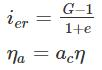η = percentage air voids

ac = air content

η = porosity

calculation:

icr = iper x FOS

icr = 0.315 × 3

icr = 0.945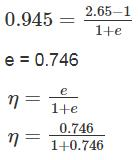η = 0.427 = 42.7%

ac = 15%

ηa = 0.427 × 0.15

ηa = 0.06405

ηa = 6.405%

QUESTION: 9

15 metres of thick saturated clay is underlain by the sand layer and the sand layer is under artesian pressure equivalent to 5 metres head of water. Excavation is started and is it done up to 12.7 metres of depth in the clay layer at which the bottom heaves. For this much excavation depth in the clay. How much-saturated unit weight of clay you expect such that bottom will not heaves?

Solution: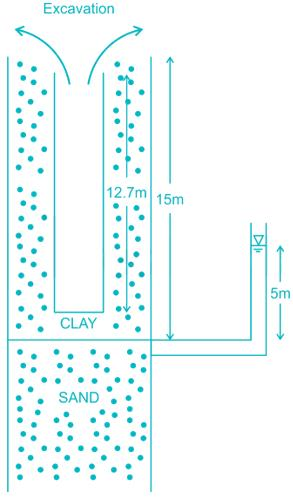At C - C

∑ FB = 0

(15 - 12.70) × γsat - 5 × 9.81 = 0

γsat = 21.33 kN/m3

*Answer can only contain numeric values
QUESTION: 10

A 50 cm well completely penetrates an artesian aquifer. The length of the strainer is 25m. Determine the discharge (in lph) from the well, when the drawdown in the pumping well is 4 m. The K of the aquifer is 45 m/day. At a radial distance of 400 m from center of well, drawdown is zero.

Solution:

For artesian (or) confined aquifer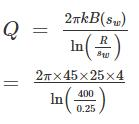= 3832.37 m3/day

= 159682.26 lph